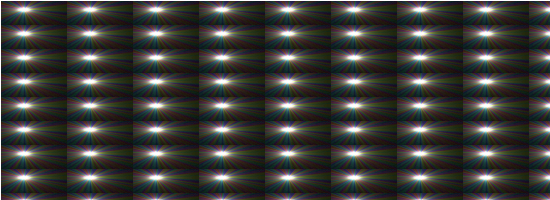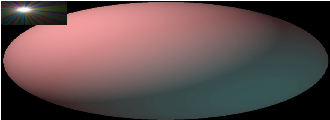# SVGBasics

## Using Filters to Add Raster Images

### Filters

Filter effects are the single most visually stunning part of SVG. I can only touch on the effects that are possible, but given a taste of the syntax, you should be able to apply methods you've learned elsewhere.
I'll only cover about half of the filter effects in the specification, and only demonstrate a few of the way to use these. The intent here is to give an idea of what can be done with SVG, not just repeat the entire specification.

### Inserting raster images using a filter

The 'feImage' element is a filter effect that creates an image from a raster graphic which can then be used as input to other filter effects. Here's an example which simply displays the image with no further filtering.

### feImage Example<?xml version="1.0" standalone="no"?>
<!DOCTYPE svg PUBLIC "-//W3C//DTD SVG 1.1//EN" "http://www.w3.org/Graphics/SVG/1.1/DTD/svg11.dtd">

<svg version = "1.1" viewBox = "0 0 1100 400">
<filter id = "i1" x = "0%" y = "0%" width = "100%" height = "100%">
</filter>
<g>
<rect x = "0" y = "0" width = "100%" height = "100%" filter = "url(#i1)"/>
</g>
</svg>

The 'feTile' filter effect can be used with an input to create a tiled pattern that fills the filter area.

### feTile Example<?xml version="1.0" standalone="no"?>
<!DOCTYPE svg PUBLIC "-//W3C//DTD SVG 1.1//EN" "http://www.w3.org/Graphics/SVG/1.1/DTD/svg11.dtd">

<svg viewBox = "0 0 1100 400" version = "1.1">
<filter id = "i1">
<feImage x = "0" y = "0" width = "10%" height = "10%" result = "raster1" xlink:href = "../images/nova.png"/>
<feTile/>
</filter>
<g>
<rect x = "0" y = "0" width = "100%" height = "100%" filter = "url(#i1)"/>
</g>
</svg>

### 'feBlend'

The feBlend effect places one image on another. To do this, it takes two images as parameters in and in2 then uses the 'mode' attribute to decide how to combine pixels from the images.
The simplest mode to understand is 'normal' which is the default. Using 'normal' will cause pixels from the first to be placed on top of those in the second. Any place where the first image is transparent, the second image will show through. This mode will get you a lot of mileage, if don't know you need one of the other modes (multiply, screen, darken, or lighten) then just use normal.

### feBlend Example<?xml version="1.0" standalone="no"?>
<!DOCTYPE svg PUBLIC "-//W3C//DTD SVG 1.1//EN" "http://www.w3.org/Graphics/SVG/1.1/DTD/svg11.dtd">

<svg viewBox = "0 0 1100 400" version = "1.1">
<filter id = "i1">
<feImage x = "0" y = "0" width = "10%" height = "10%" result = "raster1" xlink:href = "../images/nova.png"/>
<feTile result = "tile1"/>
<feImage x = "0" y = "0" width = "50%" height = "50%" result = "raster2" xlink:href = "../images/sphere1.png"/>
<feBlend in = "raster1" in2 = "raster2"/>
</filter>
<g>
<rect x = "0" y = "0" width = "100%" height = "100%" filter = "url(#i1)"/>
</g>
</svg>

### 'feComposite'

The feBlend effect places one image on another. To do this, it takes two images as parameters in and in2 then uses the 'mode' attribute to decide how to combine pixels from the images.
The simplest mode to understand is 'normal' which is the default. Using 'normal' will cause pixels from the first to be placed on top of those in the second. Any place where the first image is transparent, the second image will show through. This mode will get you a lot of mileage, if don't know you need one of the other modes (multiply, screen, darken, or lighten) then just use normal.

### feComposite Example<?xml version="1.0" standalone="no"?>
<!DOCTYPE svg PUBLIC "-//W3C//DTD SVG 1.1//EN" "http://www.w3.org/Graphics/SVG/1.1/DTD/svg11.dtd">

<svg viewBox = "0 0 1100 400" version = "1.1">
<filter id = "i1">
<feImage x = "0" y = "0" width = "10%" height = "10%" result = "raster1" xlink:href = "../images/nova.png"/>
<feTile result = "tile1"/>
<feImage x = "0" y = "0" width = "50%" height = "50%" result = "raster2" xlink:href = "../images/sphere1.png"/>
<feComposite in = "raster1" in2 = "raster2"/>
</filter>
<g>
<rect x = "0" y = "0" width = "100%" height = "100%" filter = "url(#i1)"/>
</g>
</svg>

### 'feMerge'

The feBlend effect places one image on another. To do this, it takes two images as parameters in and in2 then uses the 'mode' attribute to decide how to combine pixels from the images.
The simplest mode to understand is 'normal' which is the default. Using 'normal' will cause pixels from the first to be placed on top of those in the second. Any place where the first image is transparent, the second image will show through. This mode will get you a lot of mileage, if don't know you need one of the other modes (multiply, screen, darken, or lighten) then just use normal.

### feMerge Example<?xml version="1.0" standalone="no"?>
<!DOCTYPE svg PUBLIC "-//W3C//DTD SVG 1.1//EN" "http://www.w3.org/Graphics/SVG/1.1/DTD/svg11.dtd">

<svg viewBox = "0 0 1100 400" version = "1.1">
<filter id = "i1">
<feImage x = "0" y = "0" width = "10%" height = "10%" result = "raster1" xlink:href = "../images/nova.png"/>
<feTile result = "tile1"/>
<feImage x = "0" y = "0" width = "50%" height = "50%" result = "raster2" xlink:href = "../images/sphere1.png"/>
<feMerge>
<feMergeNode in = "raster2"/>
<feMergeNode in = "raster1"/>
</feMerge>
</filter>
<g>
<rect x = "0" y = "0" width = "100%" height = "100%" filter = "url(#i1)"/>
</g>
</svg>